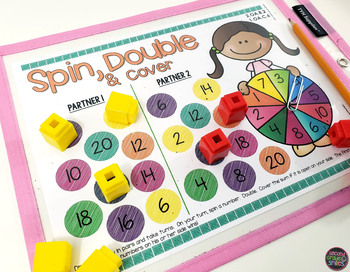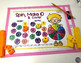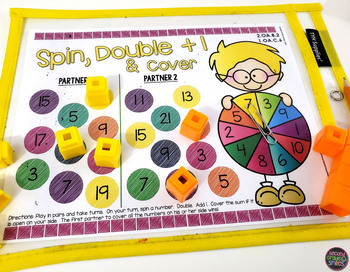Addition and Subtraction Games | Fact Fluency GamesSubject
Resource Type
Common Core State Standards
Product Rating
File Type

PDF (Acrobat) Document File

Be sure that you have an application to open this file type before downloading and/or purchasing.

7 MB|28 pages
Share
Also included in:
1. Includes nine of my partner spin and write and wipe math game resources in a money-saving bundle. These games are perfect for math centers and math stations! They encourage cooperative learning, are self-checking, and require very little teacher prep! Just print, place in a page protector, and add s
\$39.00
\$30.00
Save \$9.00
Product Description

This set of addition and subtraction games includes 12 self-checking, partner spin and write and wipe games for independent or guided fact fluency practice! Use them for early finishers, math tubs, or math centers. Perfect for developing fact fluency in grade 1 and grade 2!

---

The following games are included in both color and black and white versions (the black and white versions work great as homework activities or as a summer send home pack!):

Spin, Double & Cover

Spin, Double +1 & Cover

Spin, Double -1 & Cover

Spin It and Turn It Around (Turn Around Facts)

Spin a Family of Facts (with sums 10 and under)

Spin a Family of Facts (with sums up to 20)

Spin, Make 10 & Cover

Spin, Make 20 & Cover

Spin, Add 9 & Cover

Spin, Subtract 9 & Cover

Balance That Addition Equation

Balance That Subtraction Equation

Prep is easy! Print the desired game boards and laminate or place in a page protector. Provide dry erase markers and basic manipulatives as indicated in the instructions. These may include counters or paperclips for use as spinners.

---

These activities address the following Common Core Standards:

CCSS.MATH.CONTENT.1.OA.B.3: Apply properties of operations as strategies to add and subtract.2 Examples: If 8 + 3 = 11 is known, then 3 + 8 = 11 is also known. (Commutative property of addition.) To add 2 + 6 + 4, the second two numbers can be added to make a ten, so 2 + 6 + 4 = 2 + 10 = 12. (Associative property of addition.)

CCSS.MATH.CONTENT.1.OA.C.6: Add and subtract within 20, demonstrating fluency for addition and subtraction within 10. Use strategies such as counting on; making ten (e.g., 8 + 6 = 8 + 2 + 4 = 10 + 4 = 14); decomposing a number leading to a ten (e.g., 13 - 4 = 13 - 3 - 1 = 10 - 1 = 9); using the relationship between addition and subtraction (e.g., knowing that 8 + 4 = 12, one knows 12 - 8 = 4); and creating equivalent but easier or known sums (e.g., adding 6 + 7 by creating the known equivalent 6 + 6 + 1 = 12 + 1 = 13).

CCSS.MATH.CONTENT.1.OA.D.7: Understand the meaning of the equal sign, and determine if equations involving addition and subtraction are true or false. For example, which of the following equations are true and which are false? 6 = 6, 7 = 8 - 1, 5 + 2 = 2 + 5, 4 + 1 = 5 + 2.

CCSS.MATH.CONTENT.1.OA.D.8: Determine the unknown whole number in an addition or subtraction equation relating three whole numbers. For example, determine the unknown number that makes the equation true in each of the equations 8 + ? = 11, 5 = _ - 3, 6 + 6 = _.

CCSS.MATH.CONTENT.2.OA.B.2: Fluently add and subtract within 20 using mental strategies.2 By end of Grade 2, know from memory all sums of two one-digit numbers.

*Standards are also printed on each game board for easy reference.*

---

Related Products

---

If you and your students enjoy this resource, please consider following my store. Freebies and new products are posted regularly. You can contact me with any questions or requests at secondgradesmilesstore@gmail.com.

- Amanda Taylor @ Second Grade Smiles

Total Pages
28 pages
N/A
Teaching Duration
Lifelong tool
Report this Resource to TpT
Reported resources will be reviewed by our team. Report this resource to let us know if this resource violates TpT’s content guidelines.